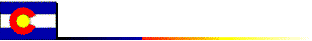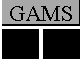## GAMS Utilities for Domain Extraction

### March, 2005

* This research supported by the GAMS Applied General Equilibrium Research Fund. The author remains responsible for any bugs which exist in this software. This software is not officially supported by GAMS Corporation.

A "tuple" in GAMS is a logical mapping between combinations of two or more sets. A common problem in GAMS programming is to construct tuples at execution time which correspond to the nonzero pattern of data in a parameter or variable. Here is a simple example to illustrate the reason this is important. Suppose that you want to write a CSV file based on the data in a parameter x(i,j,k) where the sets i, j and k are large and many of the elements of the parameter x are zero. If you write the following construct, your end up with many rows of zeros in your output:
```
loop((i,j), put i.tl,j.tl; loop(k, put x(i,j,k)); put /;);

```
Another way you might do this is to place a restriction on the outer loop to suppress rows of zeros, for example:
```
loop((i,j)\$(sum(k,abs(x(i,j,k)) > eps),
put i.tl,j.tl; loop(k, put x(i,j,k)); put /;);

```
The problem with this approach is execution speed. If the sets are several thousands of elements each you can end up waiting for hours to generate your output file.

The new routines for domain extraction operate very quickly and provide a nice clean solution. In this case we use the rdomain call to extract the "row domain" of parmaeter x:

```
set	rows(i,j), cols(k);

\$libinclude rdomain x rows cols

loop(rows(i,j),	put i.tl,j.tl; loop(cols(k), put x(i,j,k)); put /;);

```
The rdomain routine efficiently determines the set of tuples over sets i and j in which there are nonzero elements of parameter x.

Three GAMS libinclude routines are provided which return sets and tuples corresponding to the non-zero structure of GAMS numeric arrays (parameters, variables or equations).

The routines included here include:

• domain returns the nonzero domain of a given parameter. For example:
```
parameter	x(i,j,k)	A sparse matrix;

... GAMS statements which assign values to X

set		ix(i,j,k)	Nonzeros in X;

\$libinclude domain x ix

```
• rdomain returns the nonzero "row domain" of a given parameter. For example:
```
parameter	x(i,j,k)	A sparse matrix;

... GAMS statements which assign values to X

set		ir(i,j)		Nonzero rows in X,
ic(k)		Nonzero columns in X;

\$libinclude rdomain x ir ic

```
• cdomain returns the nonzero "component domain" of a given parameter. For example:
```
parameter	x(i,j,k)	A sparse matrix;

... GAMS statements which assign values to X

set	ii(i)		Nonzeros in the first component of X
jj(k)		Nonzeros in the second component of X
kk(k)		Nonzeros in the third component of X

\$libinclude cdomain x ii jj kk
```
These routines exploit a powerful but somewhat cryptic option statement which has been introduced recently to the GAMS compiler. These routines therefore require a GAMS system which has been released after November, 2004.

### Contents

#### Installation:

N.B. If you wish to use these utilities in GAMS programs which will be distributed to others, you may include the utilities in your model directory and call them using \$batinclude rather than \$libinclude.

#### Example 1: Test problems for \$libinclude domain.

```
*	Interrupt the program if the GAMS compiler is out of date:

\$version 139

*	Turn on profiling to track execution time:

option profile=1;

*	Declare and populate some sparse parameters:

set	i/i1*i10/;
alias (i,i1,i2,i3,i4,i5,i6,i7,i8,i9,j);
parameter
x1(i1)
x2(i1,i2),
x3(i1,i2,i3),
x4(i1,i2,i3,i4),
x5(i1,i2,i3,i4,i5),
x6(i1,i2,i3,i4,i5,i6);
x1(j)\$(uniform(0,1) > 0.99) = uniform(0,1);
x2(i1,j)\$(uniform(0,1) > 0.99) = uniform(0,1);
x3(i1,i2,j)\$(uniform(0,1) > 0.99) = uniform(0,1);
x4(i1,i2,i3,j)\$(uniform(0,1) > 0.99) = uniform(0,1);
x5(i1,i2,i3,i4,j)\$(uniform(0,1) > 0.99) = uniform(0,1);
x6(i1,i2,i3,i4,i5,j)\$(uniform(0,1) > 0.99) = uniform(0,1);

*	Declare sets which can be used to hold the nonzero
*	domains:

set	d1(i1),
d2(i1,i2),
d3(i1,i2,i3),
d4(i1,i2,i3,i4),
d5(i1,i2,i3,i4,i5),
d6(i1,i2,i3,i4,i5,i6);

\$libinclude domain x1 d1
\$libinclude domain x2 d2
\$libinclude domain x3 d3
\$libinclude domain x4 d4
\$libinclude domain x5 d5
\$libinclude domain x6 d6

display	d1,d2,d3,d4,d5,d6;

```

#### Example 2: Test problems for \$libinclude rdomain.

```\$version 139
option profile=1;
set	i/i1*i10/;
alias (i,i1,i2,i3,i4,i5,i6,i7,i8,i9,j);
parameter
x2(i1,i2),
x3(i1,i2,i3),
x4(i1,i2,i3,i4),
x5(i1,i2,i3,i4,i5),
x6(i1,i2,i3,i4,i5,i6);
x2(i1,j)\$(uniform(0,1) > 0.99) = uniform(0,1);
x3(i1,i2,j)\$(uniform(0,1) > 0.99) = uniform(0,1);
x4(i1,i2,i3,j)\$(uniform(0,1) > 0.99) = uniform(0,1);
x5(i1,i2,i3,i4,j)\$(uniform(0,1) > 0.99) = uniform(0,1);
x6(i1,i2,i3,i4,i5,j)\$(uniform(0,1) > 0.99) = uniform(0,1);

set	rd2(i1),rd3(i1,i2),rd4(i1,i2,i3),rd5(i1,i2,i3,i4),
rd6(i1,i2,i3,i4,i5),rd7(i1,i2,i3,i4,i5,i6),
cd2(i2),cd3(i3),cd4(i4),cd5(i5),cd6(i6),cd7(i7);

\$libinclude rdomain x2 rd2 cd2
\$libinclude rdomain x3 rd3 cd3
\$libinclude rdomain x4 rd4 cd4
\$libinclude rdomain x5 rd5 cd5
\$libinclude rdomain x6 rd6 cd6

display	rd2,rd3,rd4,rd5,rd6,cd2,cd3,cd4,cd5,cd6;
```

#### Example 3: Test problems for \$libinclude cdomain.

```\$version 139

option profile=1;
set	i/i1*i10/;
alias (i,i1,i2,i3,i4,i5,i6,i7,i8,i9,j);
parameter
x2(i1,i2),
x3(i1,i2,i3),
x4(i1,i2,i3,i4),
x5(i1,i2,i3,i4,i5),
x6(i1,i2,i3,i4,i5,i6);
x2(i1,j)\$(uniform(0,1) > 0.99) = uniform(0,1);
x3(i1,i2,j)\$(uniform(0,1) > 0.99) = uniform(0,1);
x4(i1,i2,i3,j)\$(uniform(0,1) > 0.99) = uniform(0,1);
x5(i1,i2,i3,i4,j)\$(uniform(0,1) > 0.99) = uniform(0,1);
x6(i1,i2,i3,i4,i5,j)\$(uniform(0,1) > 0.99) = uniform(0,1);

set	j1(i),j2(i),j3(i),j4(i),j5(i),j6(i),j7(i);

\$libinclude cdomain x2 j1 j2
display j1, j2;

\$libinclude cdomain x3 j1 j2 j3
display j1, j2, j3;

\$libinclude cdomain x4 j1 j2 j3 j4
display j1, j2, j3, j4;

\$libinclude cdomain x5 j1 j2 j3 j4 j5
display j1, j2, j3, j4, j5;

\$libinclude cdomain x6 j1 j2 j3 j4 j5 j6
display j1, j2, j3, j4, j5, j6;
```